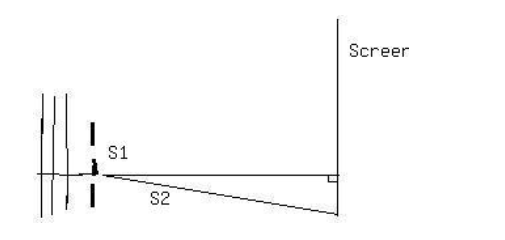Ask question

# A line passes through (9,3),(12,4), and (n,-5) Find the value of n.# A line passes through (9,3),(12,4), and (n,-5) Find the value of n.

Question
Vectors and spacesasked 2020-10-26
A line passes through (9,3),(12,4), and (n,-5)
Find the value of n.

## Answers (1)2020-10-27
Given that a line passes through the points (9, 3), (12, 4), and (n, -5).
First we find the equation of the line that. passes through the points (9,3), and (12,4).
The equation of a line passing through the points $$(x_{1}, y_{1}), (x_{2}, y_{2})$$ is
$$\frac{y-y_{1}}{x-x_{1}}=\frac{y_{1}-y_{2}}{x_{1}-x_{2}}$$
In this problem $$(x_{1}, y_{1}) = (9, 3),\ and\ (x_{2}, y_{2}) = (12,4).$$
This implies that $$x_{1} = 9, y_{1} = 3, x_{2} = 12, y_{2} =4$$
. Plugging these values $$\in \frac{y-y_{1}}{x-x_{1}}=\frac{y_{1}-y_{2}}{x_{1}-x_{2}}$$ we get the equation of the line is
$$\in \frac{y-y_{1}}{x-x_{1}}=\frac{y_{1}-y_{2}}{x_{1}-x_{2}}$$
$$\Rightarrow \frac{y-3}{x-9}=\frac{3-4}{9-12}$$
$$\Rightarrow \frac{y-3}{x-9}=\frac{1}{3}$$
$$\Rightarrow 3(y - 3) = x - 9$$
$$\Rightarrow 3y - 9 = x - 9$$
$$\Rightarrow x - 3y = 0$$
Therefore, the equation of the line is $$x - 3y = 0$$
Also given that the line passing through the point $$(n, -5)$$
Putting $$x = n,\ and\ y = -5$$ in the equation of the line we get
$$x - 3y = 0$$
$$\Rightarrown - 3(-5) = 0$$
$$\Rightarrow n + 15 = 0$$
$$\Rightarrow n = -15$$
Thus, the value of n is -15,

### Relevant Questionsasked 2021-03-01
Write an equation of the line that passes through (3, 1) and (0, 10)asked 2021-05-181. S1 and S2, shown above, are thin parallel slits in an opaqueplate. A plane wave of wavelength λ is incident from the leftmoving in a direction perpendicular to the plate. On a screenfar from the slits there are maximums and minimums in intensity atvarious angles measured from the center line. As the angle isincreased from zero, the first minimum occurs at 3 degrees. Thenext minimum occurs at an angle of-
A. 4.5 degrees
B. 6 degrees
C. 7.5 degrees
D. 9 degrees
E. 12 degreesasked 2020-10-18
Find the vector and parametric equations for the line through the point P=(5,−2,3) and the point Q=(2,−7,8).asked 2020-11-16
Find the vector and parametric equations for the line through the point P=(5,−2,3) and the point Q=(2,−7,8).asked 2021-03-08
The position vector $$\displaystyle{r}{\left({t}\right)}={\left\langle{n}{t},\frac{{1}}{{t}^{{2}}},{t}^{{4}}\right\rangle}$$ describes the path of an object moving in space.
(a) Find the velocity vector, speed, and acceleration vector of the object.
(b) Evaluate the velocity vector and acceleration vector of the object at the given value of $$\displaystyle{t}=\sqrt{{3}}$$asked 2021-01-31

The quadratic function $$\displaystyle{y}={a}{x}^{2}+{b}{x}+{c}$$ whose graph passes through the points (1, 4), (2, 1) and (3, 4).asked 2021-05-03
A charge of $$\displaystyle{6.00}\times{10}^{{-{9}}}$$ C and a charge of $$\displaystyle-{3.00}\times{10}^{{-{9}}}$$ C are separated by a distance of 60.0 cm. Find the position at which a third charge, of $$\displaystyle{12.0}\times{10}^{{-{9}}}$$ C, can be placed so that the net electrostatic force on it is zero.asked 2021-05-16
Consider the curves in the first quadrant that have equationsy=Aexp(7x), where A is a positive constant. Different valuesof A give different curves. The curves form a family,F. Let P=(6,6). Let C be the number of the family Fthat goes through P.
A. Let y=f(x) be the equation of C. Find f(x).
B. Find the slope at P of the tangent to C.
C. A curve D is a perpendicular to C at P. What is the slope of thetangent to D at the point P?
D. Give a formula g(y) for the slope at (x,y) of the member of Fthat goes through (x,y). The formula should not involve A orx.
E. A curve which at each of its points is perpendicular to themember of the family F that goes through that point is called anorthogonal trajectory of F. Each orthogonal trajectory to Fsatisfies the differential equation dy/dx = -1/g(y), where g(y) isthe answer to part D.
Find a function of h(y) such that x=h(y) is the equation of theorthogonal trajectory to F that passes through the point P.asked 2021-01-30

A line L through the origin in $$\displaystyle\mathbb{R}^{{3}}$$ can be represented by parametric equations of the form x = at, y = bt, and z = ct. Use these equations to show that L is a subspase of $$RR^3$$  by showing that if $$v_1=(x_1,y_1,z_1)\ and\ v_2=(x_2,y_2,z_2)$$  are points on L and k is any real number, then $$kv_1\ and\ v_1+v_2$$  are also points on L.asked 2020-10-21
Find the Euclidean distance between u and v and the cosine of the angle between those vectors. State whether that angle is acute, obtuse, or $$\displaystyle{90}^{{\circ}}$$. u = (-1, -1, 8, 0), v = (5,6,1,4)
...